Subsections

# Virial Expansion

## Mayer Function

For ideal gas U=0. Let us discuss slightly non-ideal gas, and expand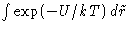when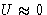.

Simple idea:
Expand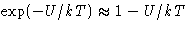Problem:
Since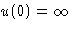, this never works!
Solution:
Let us discuss Mayer function instead: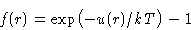(3)
When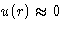Mayer function is small. When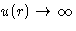we have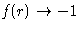.
Assumption:
For slightly non-ideal gas Mayer function is small on average: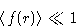## Partition Function and Free Energy

Gibbs factor: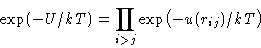Express through Mayer function:Expand: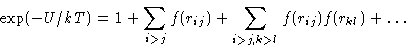(4)

When is f(r) is non-zero? For hard spheres--when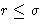. This is collision. Low density gas--not many collisions! Take a volume V, where collisions are rare. It means neglecting products of f-functions.

To obtain ZN, integrate (4) over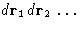1.
First term:2.
Second term: a collection of equal contributions likeNumber of terms: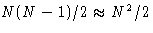(a)
Integration over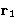--factor V
(b)
Integration over--factor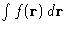(c)
Integration over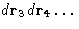--factor VN-2

Result: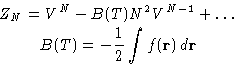B(T) is second virial coefficient

Taking log: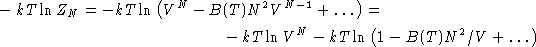Since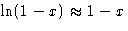, we obtained:Pressure: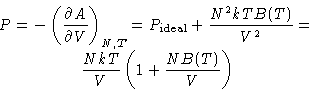(5)

## Second Virial Coefficient

If we know u(r), we can calculate B!

Dimension:
Since f(r) is dimensionless, B has dimension of volume.
Model:
Hard core plus attraction, equation (2).
High Temperatures:
If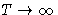, Mayer function is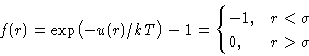and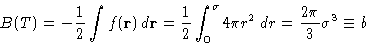This is four times the volume of one molecule
At high temperatures B(T)=b describes the hard core volume of gas.
Lower Temperatures:
Integrate from to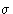and fromto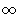. First integral gives b, second is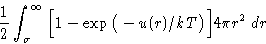Expansion: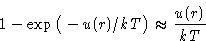and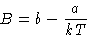(6)
with positive a (at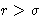potential u is attractive!)Assumption:
We assume (6) is valid for all temperatures.
Meaning of the coefficients:
b describes steric repulsion, a describes long range attraction.
Behavior of B(T):
• At high T we have B(T)>0 (repulsion)
• At lower T we have B(T)<0 (attraction)
• At some temperature Tb it is zero B(Tb)=0. This is Boyle point of gas.

## Higher Order Terms

### Expansion

If we include the terms fikflm in expansion ZN, we will obtain: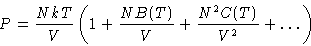(7)
C(T) is the third virial coefficient. Similarly fourth, fifth....

We can compute any virial coefficient if we know u(r)!

### Convergence

Will these series converge? Yes, if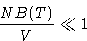It means that distances between moleculestheir sizes!

This is true for gases, but not for liquids!

Virial expansion does not work for liquids.Next: Van der Waals Equation Up: Imperfect Gases and Liquids. Previous: Interaction Energy

© 1997 Boris Veytsman and Michael Kotelyanskii
Thu Sep 18 22:50:29 EDT 1997